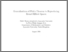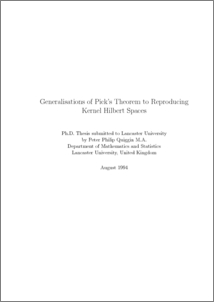# Generalisations of Pick's theorem to reproducing Kernel Hilbert spaces

Quiggin, Peter Philip (1994) Generalisations of Pick's theorem to reproducing Kernel Hilbert spaces. PhD thesis, UNSPECIFIED.Preview
PDF
Quiggin.pdf - Published Version

## Abstract

Pick's theorem states that there exists a function in H1, which is bounded by 1 and takes given values at given points, if and only if a certain matrix is positive. H1 is the space of multipliers of H2 and this theorem has a natural generalisation when H1 is replaced by the space of multipliers of a general reproducing kernel Hilbert space H(K) (where K is the reproducing kernel). J. Agler showed that this generalised theorem is true when H(K) is a certain Sobolev space or the Dirichlet space. This thesis widens Agler's approach to cover reproducing kernel Hilbert spaces in general and derives sucient (and usable) conditions on the kernel K, for the generalised Pick's theorem to be true for H(K). These conditions are then used to prove Pick's theorem for certain weighted Hardy and Sobolev spaces and for a functional Hilbert space introduced by Saitoh. The reproducing kernel approach is then used to derived results for several related problems. These include the uniqueness of the optimal interpolating multiplier, the case of operator-valued functions and a proof of the Adamyan-Arov-Kren theorem.

Item Type:
Thesis (PhD)
Departments:
ID Code:
61962
Deposited By:
Deposited On:
24 Jan 2013 16:28
Refereed?:
No
Published?:
Published